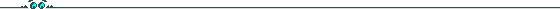# School on Singularities in Algebraic Geometry & String Theory

## July 8-17, 1999 ``` ``` COURSES (Detailed)

```

``````
```

### Paul Aspinwall

#### Singularities and String Duality

```
```
• Riemannian manifolds and the sigma model.
• Orbifolds and the DHVW formula
• The B-field
• Mirror symmetry and the moduli space of complexified Kaehler forms.
• Flops
• The Cartan-Leray spectral sequence and discrete torsion
• Duality and Du Val singularities on a K3 surface
• Representation theory on Calabi-Yau threefolds and anomaly cancellation
```

``````

```

### Victor Batyrev

#### Introduction to Toric Varieties and Mirror Symmetry

```
```
• Linear actions of algebraic tori on affine spaces. Toric varieties as spaces of orbits
• Toric varieties as equivariant compactifications of algebraic tori
• Singularities of toric varieties and their resolution. Toric flip and flop
• Hypersurfaces in toric varieties and their singularities. Calabi-Yau hypersurfaces
• Reflexive polyhedra and mirror duality
```

``````
```

### Philip Candelas

#### String Dualities and Toric Geometry

```
```
• What is string theory?
• String dualities
• Correspondence between vector bundles on K3 and CY 3-folds
• CY manifolds over finite fields
```

``````

```

### Le Dung-Trang

#### Introduction to Singularities

```
```
• Definition of an algebraic singularity
• Plane curve singularities
• Introduction to hypersurface singularities
• Introduction to general singularities
```

``````
```

### M.S. Narasimhan

#### Moduli Spaces of Vector and G-bundles over Riemann Surfaces

```
```
• Geometric invariant theory
• Construction of moduli spaces
• Singularities of the moduli spaces
• The theta line bundle and the Picard group
• Moduli spaces of principal G-bundles
```

``````
```

### Miles Reid

#### Resolution of Quotient Singularities and McKay Correspondence

```
```
Abstract: Let G in GL(n) be a finite group acting on CC^n, and consider the quotient space X = CC^n/G and a resolution (or partial resolution) Y -> X. The question behind the McKay correspondence is to ask for a relation between the geometry of Y and the group representation theory of G. For G in SL(2) and SL(3), and Y a minimal (or "crepant") resolution Y, this can be done in some detail in several quite different ways. The lectures will describe quotient singularities and their resolution, the background to McKay correspondence, several of the known approaches to proving the McKay correspondence, and many open problems arising.

Approximate program for the lectures:

• Background
• The ramification approach (Ito--Reid)
• The stringy motive approach (Batyrev, Kontesvich, Denef and Loeser)
• Hilbert schemes as crepant resolutions (Nakamura)
• The derived category approach to McKay (Ito--Nakajima, Orlov, Bridgeland and King)
• Compatibility between the different approaches, and other open problems
```

```